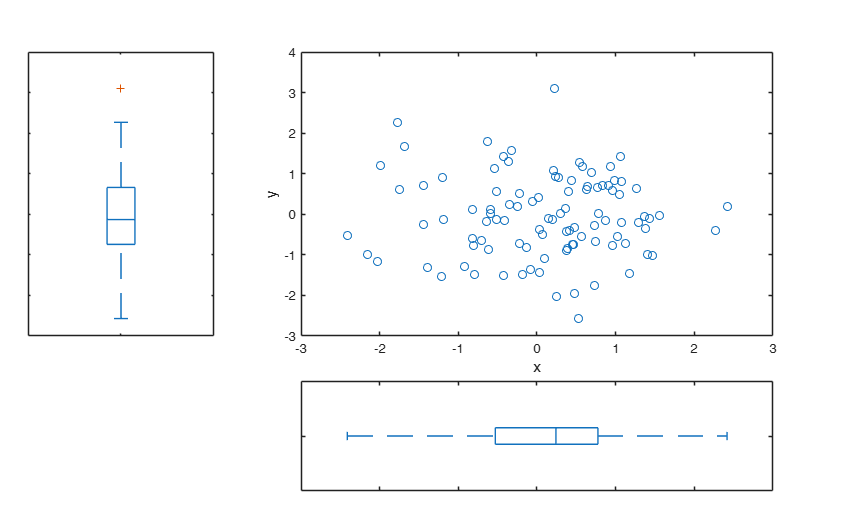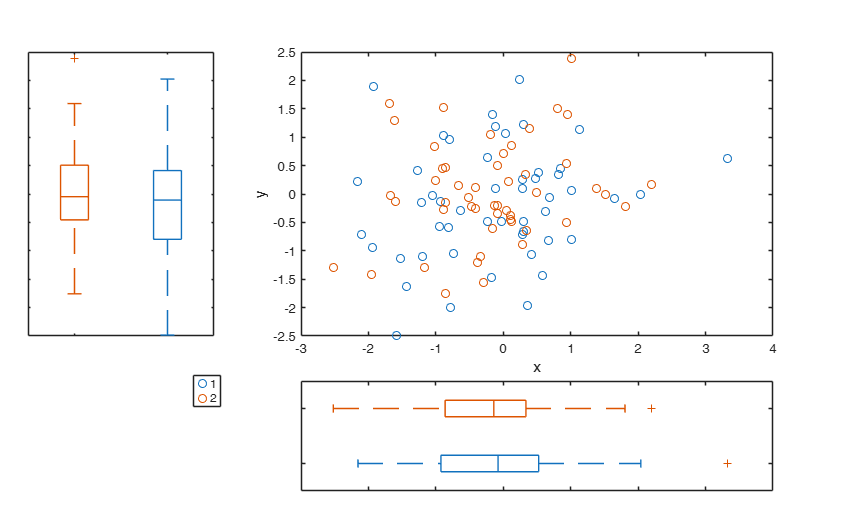# scatterboxplot

scatterboxplot creates scatter diagram with marginal boxplots

## Syntax

• h=scatterboxplot(x,y)example
• h=scatterboxplot(x,y,Name,Value)example

## Description

scatterboxplot displays a 2D scatter plot with marginal boxplots. It receives data in two vectors X and Y, and puts a univariate boxplot on the horizontal and vertical axes of the plot. x and y must have the same length. All the name pairs in function scatterhist such as 'Location', 'Group', 'PlotGroup',... can be used inside this function.

 h =scatterboxplot(x, y) A 2D scatter plot with marginal boxplots.

 h =scatterboxplot(x, y, Name, Value) A 2D scatter plot with marginal boxplots, for grouped data.

## Examples

expand all

###A 2D scatter plot with marginal boxplots.

n=100;
scatterboxplot(randn(n,1),randn(n,1));###A 2D scatter plot with marginal boxplots, for grouped data.

n=100; group=ones(n,1);
group(1:50)=2; scatterboxplot(randn(n,1),randn(n,1),'Group',group);## Related Examples

expand all

###scatterboxplot for the stars data.

Y=load('stars.txt');
x=Y(:,1); y=Y(:,2);
out=FSR(y,x,'plots',0);
group=ones(length(x),1);
group(out.outliers)=2;
scatterboxplot(Y(:,1),Y(:,2),'Group',group);
-------------------------
Signal detection loop
Signal in final part of the search: step 43 because
rmin(43,47)>99% at final step: Bonferroni signal in the final part of the search.
rmin(43,47)>99.999%
------------------------------------------------
-------------------
Signal validation exceedance of upper envelopes
Validated signal
-------------------------------
Start resuperimposing envelopes from step m=42
Superimposition stopped because r_{min}(43,44)>99% envelope
$r_{min}(43,44)>99$\% envelope
----------------------------
Final output
Number of units declared as outliers=4
Summary of the exceedances
1          99         999        9999       99999
0           2           2           1           1## Input Arguments

### x — Input data. Vector or 1-column matrix.

x contains the univariate data to display in the x axis.

Data Types: single| double

### y — Input data. Vector or 1-column matrix.

y contains the univariate data to display in the y axis.

Data Types: single| double

### Name-Value Pair Arguments

Specify optional comma-separated pairs of Name,Value arguments. Name is the argument name and Value is the corresponding value. Name must appear inside single quotes (' '). You can specify several name and value pair arguments in any order as  Name1,Value1,...,NameN,ValueN.

Example: ,

### Group —A grouping variable.vector.

Vector of group identifiers.

With this option scatterboxplot creates a 2D GSCATTER plot instead of a SCATTER plot, and the marginal boxplots are replaced by grouped boxplots.

Data Types - categorical array | logical or numeric vector | character array | string array | cell array of character vectors

Example:

Data Types: categorical array | logical or numeric vector | character array | string array | cell array of character vectors Example - 'Group',[1,1,1,2,2,2,2,2,2]

### PlotGroup —Grouped plot indicator.character.

If PlotGroup is 'on' routine displays grouped boxplots.

This is the default if a Group parameter is specified.

If PlotGroup is 'off' scatterboxplot displays boxplots of the whole data set. This is the default if a Group parameter is not specified.

Data Types - character 'on' or 'off'

Example:

Data Types: character 'on' or 'off' Example - 'PlotGroup','on'

## Output Arguments

### h —Vector of handles.  Vector

Contains the three axes handles for the scatterplot, the plot along the horizontal axis, and the plot along the vertical axis, respectively..

Data Types - single | double.